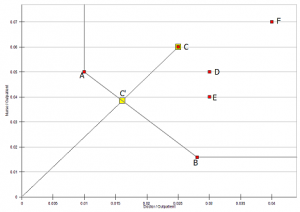Skip to toolbar

## Convex hull

The convex hull for a set of units (represented as points) is defined as the smallest convex polygon that encompasses all the points and is a further restriction on a convex cone. The envelopment surface specified by the BCC model results in a convex hull. See also: convex one.

## Convex cone – Conical hull

A convex cone has its vertex as its origin and includes all nonnegtive linear combinations of its points. The envelopment surface obtained from the CCR model has the shape of a convex cone. If the situation in Figure 1 were drawn 3-dimensionally, the efficiency frontier would consist of a set of interlaced facets formed by hyperplanes emanating from the origin and forming a conical structure. The efficient units would lie on top of the facets, while the inefficient ones would be covered under the cone. For a clear visual representation, refer to Ah and Seiford (1993).
-Ah A and Seiford L (1993), ‘The mathematical programming approach to efficiency analysis’, in: Fried H, Knox C A K and Schmidt S (editors), The measurement of productive efficiency: techniques and applications, Oxford University Press.

## Constant returns to scale

unit operates under constant returns to scale if an increase in inputs results in a proportionate increase in the output levels. If the inputs values for a unit are all doubled, then the unit must produce twice as much outputs. In a single input and output case, the efficiency frontier reduces to a straight line. See also: variable returns to scale.

## Decreasing returns to scale

A unit is said to operate at decreasing returns to scale (DRS) if a proportionate increase in all of its inputs results in a less than proportionate increase in its outputs. If for a given DMU, the sum of the dual weights in the dual model (M2) is greater than 1, then that unit can be said to operate at DRS, assuming it is technically efficient. See Banker and Morey (1986) or Banker and Thrall (1992) for an in-depth analysis.
See also: increasing returns to scale.

– Banker R D and Morey R (1986) ‘The use of categorical variables in data envelopment analysis’, Mgmt. Sci., 32, pp 1613-1627.

-Banker R D and Thrahl R M (1992) ‘Estimation of returns to scale using data envelopment analysis’, Eur. J. of OpI. Res., 62, pp 74-84.

## DEA algorithm

Although DEA models can usually be solved with a standard LP solver, it is recommended to use specifically tailored DEA algorithms for increased accuracy and speed. Such algorithms avoid the computational problems with epsilon and make use of the fact that a unit found to be inefficient can be removed from the basis as it has no effect on the efficiency of other units (Ali 1993).

## What is Data Envelopment Analysis?

Data Envelopment Analysis is a decision making tool based on linear programming for measuring the relative efficiencies of a set of comparable units. DEA is intitaly developed by Charnes, Cooper, Rhodes (1978).

## Composite unit

A hypothetical efficient unit formed from a DMU’s reference units according to the proportions indicated by the dual weights. In Figure , the hypothetical unit for E lies at E’, defined by the reference units B and C.## CCR (ratio) mode

Named after its developer Chames, Cooper and Rhodes, this is the first and fundamental DEA model, built on the notion of efficiency as defined in the classical engineering ratio. The CCR ratio model calculates an overall efficiency for the unit in which both its pure technical efficiency and scale efficiency are aggregated into a single value. The obtained efficiency is never absolute as it is always measured relative to the field. The Chames et al (1978) article marked the birth of DEA, and despite the numerous modified models that have appeared, the CCR model is still the most widely known and used of DEA models.

## Categorical variable

A factor which can assume only a predefined set of discrete values. Categorical variables are frequently used to indicate the presence or absence of an attribute. An analysis on fast-food restaurants could use a categorical variable assigned to I to indicate the presence of a drive-in window, with the value O denoting its absence. The use of categorical variables requires modifications in DEA models as indicated in Banker and Morey (1986). See also: ordinal variable.

– Banker R D and Morey R (1986) ‘The use of categorical variables in data envelopment analysis’, Mgmt. Sci., 32, pp 1613-1627.

## DEA results are biased

Generally speaking, the results are biased if they favour a particular unit and/or unfairly assess the efficiency of some inefficient units. Although the actual DEA technique can be considered fair, bias can be introduced into the analysis through the omission of a critical factor or a lack of homogeneity in the field.

goin up